Normal view

# Linear models and generalizations : least squares and alternatives / C. Radhakrishna Rao ... [et al.]

Material type:TextLanguage: English Publication details: 2008. Edition: 3rd extended edDescription: xix, 570 p. : ill. ; 25 cmISBN: 9783540742265 ; 9780387988481Subject(s): Mathematical satistics | Linear models (Statistics)DDC classification: 519.5 Online resources: Ebook Fulltext
Contents:
TOC 1. Introduction -- 2. The Simple Linear Regression Model -- 3. The Multiple Linear Regression Model -- 4. The Generalized Linear Regression Model -- 5. Exact and Stochastic Linear Restrictions -- 6. Prediction Problems in the Generalized Regression Model -- 7. Sensitivity Analysis -- 8. Analysis of Incomplete Data Sets -- 9. Robust Regression -- 10. Models for Categorical Response Variables -- Fitting Smooth Functions -- Appendix A: Matrix Algebra
Summary: "This book provides an up-to-date account of the theory and applications of linear models. The authors present a unified theory of inference from linear models and its generalizations with minimal assumptions, not only through least squares theory, but also using alternative methods of estimation and testing based on convex loss functions and general estimating equations. It can be used as a text for courses in Read more...
Tags from this library: No tags from this library for this title.
Star ratingsAverage rating: 0.0 (0 votes)
Holdings
Item type Current library Collection Call number Copy number Status Date due Barcode Item holdsE-Book
E-book
Non-fiction 519.5 LIN 2008 (Browse shelf(Opens below)) Not for loanText
Reserve Section
Non-fiction 519.5 LIN 2008 (Browse shelf(Opens below)) C-1 Not For Loan 27312Text
Circulation Section
Non-fiction 519.5 LIN 2008 (Browse shelf(Opens below)) C-2 Available 28167
Total holds: 0
##### Browsing EWU Library shelves, Shelving location: E-book Close shelf browser (Hides shelf browser)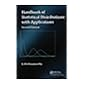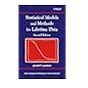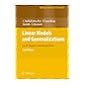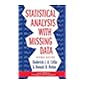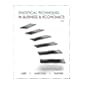519.5 KRH 2016 Handbook of statistical distributions with applications / 519.5 LAS 2003 Statistical models and methods for lifetime data / 519.5 LIB 1994 Basic statistics for business and economics / 519.5 LIN 2008 Linear models and generalizations : 519.5 LIS 2002 Statistical analysis with missing data / 519.5 LIS 2012 Statistical techniques in business & economics / 519.5 LIS 2012 Statistical techniques in business & economics /

Print version:
Linear models and generalizations.
Berlin ; New York : Springer, ©2008
(OCoLC)173807301.

Includes Bibliographical References and Index.

TOC 1. Introduction --
2. The Simple Linear Regression Model --
3. The Multiple Linear Regression Model --
4. The Generalized Linear Regression Model --
5. Exact and Stochastic Linear Restrictions --
6. Prediction Problems in the Generalized Regression Model --
7. Sensitivity Analysis --
8. Analysis of Incomplete Data Sets --
9. Robust Regression --
10. Models for Categorical Response Variables --
Fitting Smooth Functions --
Appendix A: Matrix Algebra

"This book provides an up-to-date account of the theory and applications of linear models. The authors present a unified theory of inference from linear models and its generalizations with minimal assumptions, not only through least squares theory, but also using alternative methods of estimation and testing based on convex loss functions and general estimating equations. It can be used as a text for courses in Read more...

AS

Saifun Momota

There are no comments on this title.